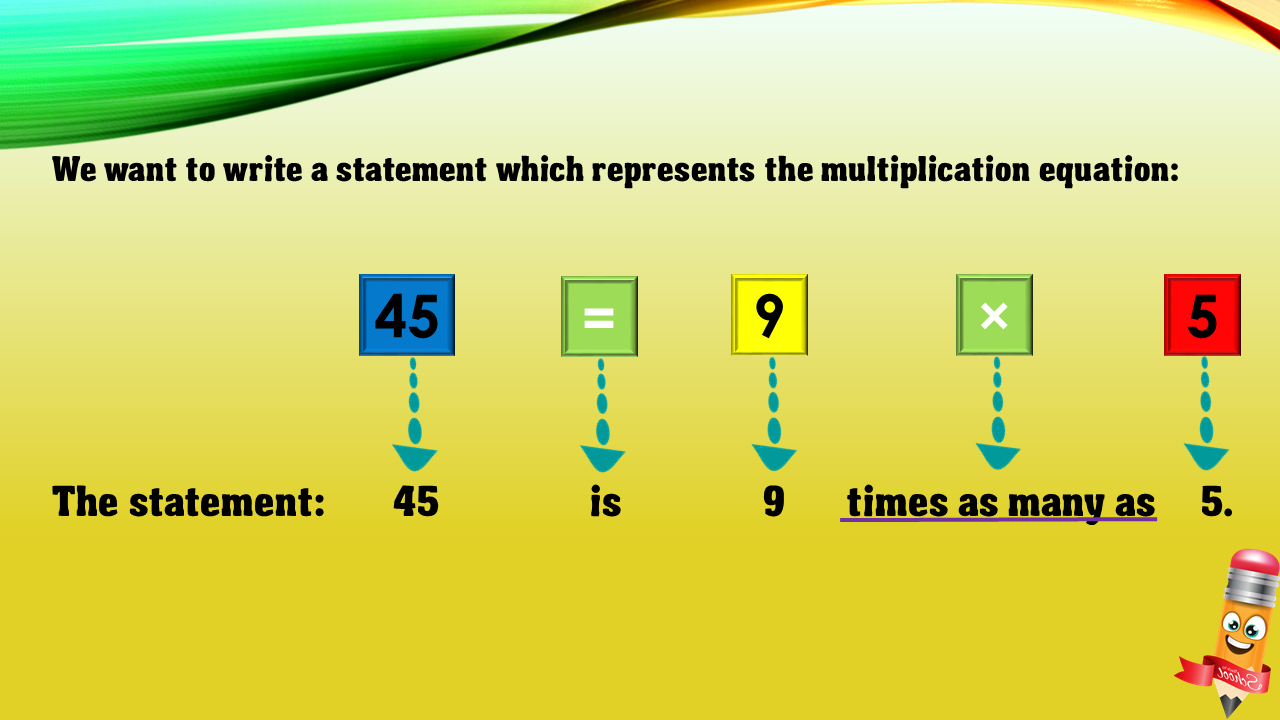1
visibility

Choose one statement which represents the equation: 45 = 9×5 ?

• A

45 is 9 more than 5.

• B

45 is 9 times as many as 5.

• C

9 is 5 less than 45.

• D

45 times as many as 9 is 5.